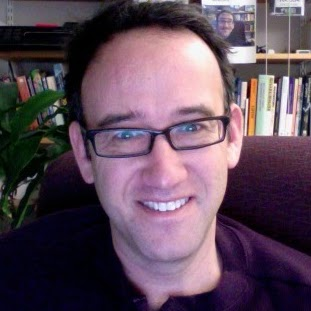# Kalman Filter

Kalman Filter is a set of mathematical equations that provides an efficient computational (recursive) means to estimate the state of a process, in a way that minimizes the mean of the squared error. It uses a series of measurements observed over time, containing noise (random variations) and other inaccuracies, and produces estimates of unknown variables that tend to be more precise than those that would be based on a single measurement alone.The filter is named for Rudolf (Rudy) E. Kálmán, one of the primary developers of its theory.

The algorithm works in a two-step process: in the prediction step, the Kalman filter produces estimates of the current state variables, along with their uncertainties. Because of the algorithm’s recursive nature, it can run in real time using only the present input measurements and the previously calculated state; no additional past information is required.

Since Kalman filter is a recursive estimator, it needs only the estimated state from the previous time step and the current measurement to compute the estimate for the current state. In contrast to batch estimation techniques, no history of observations and/or estimates is required. This can be very helpful to improve tracking of the objects. I implemented Kalman Filter in SimpleCV. As of now, I’m just predicting the center of the object using its current and previous centers. I might add more features in Kalman Filter later viz pixel velocity, real time velocity, areaRatio, etc.

Kalman Filter with OpenCV:
I tried using OpenCV 2.4 version to implement Kalman Filter, but it turns out that the bindings are incomplete. see here. As of now it’s not possible to implement Kalman Filter using cv2. So, cv it is.

Create Kalman Filter

```import cv2.cv as cv
kalman = cv.CreateKalman(4, 2, 0)
kalman_state = cv.CreateMat(4, 1, cv.CV_32FC1)
kalman_process_noise = cv.CreateMat(4, 1, cv.CV_32FC1)
kalman_measurement = cv.CreateMat(2, 1, cv.CV_32FC1)
```

Kalman filter is now ready to be used.

Set Kalman Filter

```# set previous state for prediction
kalman.state_pre[0,0]  = x
kalman.state_pre[1,0]  = y
kalman.state_pre[2,0]  = 0
kalman.state_pre[3,0]  = 0

# set kalman transition matrix
kalman.transition_matrix[0,0] = 1
kalman.transition_matrix[0,1] = 0
kalman.transition_matrix[0,2] = 0
kalman.transition_matrix[0,3] = 0
kalman.transition_matrix[1,0] = 0
kalman.transition_matrix[1,1] = 1
kalman.transition_matrix[1,2] = 0
kalman.transition_matrix[1,3] = 0
kalman.transition_matrix[2,0] = 0
kalman.transition_matrix[2,1] = 0
kalman.transition_matrix[2,2] = 0
kalman.transition_matrix[2,3] = 1
kalman.transition_matrix[3,0] = 0
kalman.transition_matrix[3,1] = 0
kalman.transition_matrix[3,2] = 0
kalman.transition_matrix[3,3] = 1

# set Kalman Filter
cv.SetIdentity(kalman.measurement_matrix, cv.RealScalar(1))
cv.SetIdentity(kalman.process_noise_cov, cv.RealScalar(1e-5))
cv.SetIdentity(kalman.measurement_noise_cov, cv.RealScalar(1e-1))
cv.SetIdentity(kalman.error_cov_post, cv.RealScalar(1))
```

Predict new points using Kalman filter

```kalman_prediction = cv.KalmanPredict(kalman)
predict_pt  = (kalman_prediction[0,0], kalman_prediction[1,0])
```

Kalman Correction

```kalman_estimated = cv.KalmanCorrect(kalman, kalman_measurement)
state_pt = (kalman_estimated[0,0], kalman_estimated[1,0])
```

Changing Kalman Measurement

```kalman_measurement[0, 0] = x
kalman_measurement[1, 0] = y
```

Pseudo Code
```Create Kalman Filter Start Tracking While (some condition)     x, y = track()     Set Kalman Filter     Change Kalman Measurements     Predict Kalman     Kalman Correction     Update the center of the object ```

This was the video where I use Kalman Filter to predict the center of the objects. I am not using Kalman Correction as of now. I have implemented Kalman Filter in SimpleCV Tracking Feature. You can find my GitHub SimpleCV Kalman branch here.

Using Kalman Filter with SimpleCV

```from SimpleCV import *
def camshift():
cam = Camera()
img = cam.getImage()
d = Display(img.size())
bb1 = getBB() # Get Bounding Box from some method
fs1=[]
while True:
try:
img1 = cam.getImage()
fs1 = img1.track("camshift",fs1,img,bb1,num_frames=5)
fs1.drawBB()
fs1.draw()
fs1.drawPredict(color=Color.RED)
camshift()
```

So, this is it. Kalman Filter.

P.S. Going back to the campus in few days. quite excited.

## 5 thoughts on “Kalman Filter”

1.Elias A. Hazboun

•jayrambhia

Hey,
I don’t exactly have an example for that. But I have included this algo in SimpleCV and here’s the link https://github.com/jayrambhia/SimpleCV/blob/develop/SimpleCV/Features/TrackSet.py#L554 . I think this might be helpful. I don’t have my laptop now but I’ll put up a code on GitHub in couple of days and drop a link here,

Cheers,
Jay

2.Abhas Bhattacharya

I was trying to use it along with face detection by using findHaarfeatures (the first binding box is supplied by it) to get a better face tracking but this tracking feature started to detect and track other parts of the skin like shoulders instead of tracking the face. Am I doing it wrong, or is the algorithm supposed to do so?

3.Simon Levy

Excellent work, Jay — thank you for this! Yours was the only code sample I could find that really showed how to do this in Python. I have bundled it into a little Python class and example for anyone who finds it useful :

4.Phillip Schmidt

Is it intended that the third 1 in the transition matrix is not on the diagonal?